JEE  >  Test: 35 Year JEE Previous Year Questions: Waves

# Test: 35 Year JEE Previous Year Questions: Waves

Test Description

## 32 Questions MCQ Test Physics For JEE | Test: 35 Year JEE Previous Year Questions: Waves

Test: 35 Year JEE Previous Year Questions: Waves for JEE 2023 is part of Physics For JEE preparation. The Test: 35 Year JEE Previous Year Questions: Waves questions and answers have been prepared according to the JEE exam syllabus.The Test: 35 Year JEE Previous Year Questions: Waves MCQs are made for JEE 2023 Exam. Find important definitions, questions, notes, meanings, examples, exercises, MCQs and online tests for Test: 35 Year JEE Previous Year Questions: Waves below.
Solutions of Test: 35 Year JEE Previous Year Questions: Waves questions in English are available as part of our Physics For JEE for JEE & Test: 35 Year JEE Previous Year Questions: Waves solutions in Hindi for Physics For JEE course. Download more important topics, notes, lectures and mock test series for JEE Exam by signing up for free. Attempt Test: 35 Year JEE Previous Year Questions: Waves | 32 questions in 60 minutes | Mock test for JEE preparation | Free important questions MCQ to study Physics For JEE for JEE Exam | Download free PDF with solutions
 1 Crore+ students have signed up on EduRev. Have you?
Test: 35 Year JEE Previous Year Questions: Waves - Question 1

### Waves y1 = A cos(0.5πx - 100πt) and y2 = A cos(0.46πx - 92πt) are travelling along x-axis. (Here x is in m and t is in second) Q. Find the number of times intensity is maximum in time interval

Detailed Solution for Test: 35 Year JEE Previous Year Questions: Waves - Question 1

The equations are y1 = A cos (0.5 πx – 100 πt) and y2 = A cos (0.46 πx – 92 πt) represents two progressive wave travelling in the same direction with slight difference in the frequency. This will give the phenomenon of beats.
Comparing it with the equation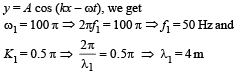Wave velocity = λ1f1 = 200 m/s  [Alternatively use v = ω/K]

ω2 = 92 π ⇒ 2πf2 = 92 π ⇒ f2 = 46 Hz Therefore beat frequency = f1 – f2 = 4 Hz and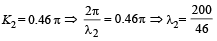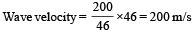NOTE : Wave velocity is same because it depends on the medium in which the wave is travelling.
Now, at x = 0, y1 + y2 = (A cos 10 πt) + (A cos 92 πt) = 0

⇒ cos 100 πt = – cos 92 πt = cos (– 92 πt)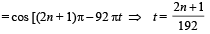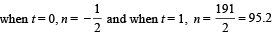⇒ net amplitude is zero for n = 96 times (the nearest answer).

Test: 35 Year JEE Previous Year Questions: Waves - Question 2

### Waves y1 = A cos(0.5πx - 100πt) and y2 = A cos(0.46πx - 92πt) are travelling along x-axis. (Here x is in m and t is in second) Q. The wave velocity of louder sound is

Detailed Solution for Test: 35 Year JEE Previous Year Questions: Waves - Question 2

The equations are y1 = A cos (0.5 πx – 100 πt) and y2 = A cos (0.46 πx – 92 πt) represents two progressive wave travelling in the same direction with slight difference in the frequency. This will give the phenomenon of beats.
Comparing it with the equationWave velocity = λ1f1 = 200 m/s  [Alternatively use v = ω/K]

ω2 = 92 π ⇒ 2πf2 = 92 π ⇒ f2 = 46 Hz Therefore beat frequency = f1 – f2 = 4 Hz andNOTE : Wave velocity is same because it depends on the medium in which the wave is travelling.
Now, at x = 0, y1 + y2 = (A cos 10 πt) + (A cos 92 πt) = 0

⇒ cos 100 πt = – cos 92 πt = cos (– 92 πt)⇒ net amplitude is zero for n = 96 times (the nearest answer).

Test: 35 Year JEE Previous Year Questions: Waves - Question 3

### Waves y1 = A cos(0.5πx - 100πt) and y2 = A cos(0.46πx - 92πt) are travelling along x-axis. (Here x is in m and t is in second) Q. The number of times y1 + y2 = 0 at x = 0 in 1 sec is

Detailed Solution for Test: 35 Year JEE Previous Year Questions: Waves - Question 3

The equations are y1 = A cos (0.5 πx – 100 πt) and y2 = A cos (0.46 πx – 92 πt) represents two progressive wave travelling in the same direction with slight difference in the frequency. This will give the phenomenon of beats.
Comparing it with the equationWave velocity = λ1f1 = 200 m/s  [Alternatively use v = ω/K]

ω2 = 92 π ⇒ 2πf2 = 92 π ⇒ f2 = 46 Hz Therefore beat frequency = f1 – f2 = 4 Hz andNOTE : Wave velocity is same because it depends on the medium in which the wave is travelling.
Now, at x = 0, y1 + y2 = (A cos 10 πt) + (A cos 92 πt) = 0

⇒ cos 100 πt = – cos 92 πt = cos (– 92 πt)⇒ net amplitude is zero for n = 96 times (the nearest answer).

Test: 35 Year JEE Previous Year Questions: Waves - Question 4

Two trains A and B moving with speeds 20 m/s and 30 m/s respectively in the same direction on the same straight track, with B ahead of A. The engines are at the front ends. The engine of train A blows a long whistle.
Assume that the sound of the whistle is composed of components varying in frequency from f1 = 800 Hz to f2 = 1120 Hz, as shown in the figure. The spread in the frequency (highest frequency – lowest frequency) is thus 320 Hz. The speed of sound in still air is 340 m/s.

Q. The speed of sound of the whistle is

Detailed Solution for Test: 35 Year JEE Previous Year Questions: Waves - Question 4

The speed of sound depends on the frame of reference of the observer.

Test: 35 Year JEE Previous Year Questions: Waves - Question 5

Two trains A and B moving with speeds 20 m/s and 30 m/s respectively in the same direction on the same straight track, with B ahead of A. The engines are at the front ends. The engine of train A blows a long whistle.
Assume that the sound of the whistle is composed of components varying in frequency from f1 = 800 Hz to f2 = 1120 Hz, as shown in the figure. The spread in the frequency (highest frequency – lowest frequency) is thus 320 Hz. The speed of sound in still air is 340 m/s.

Q. The distribution of the sound intensity of the whistle as observed by the passengers in train A is best represented by

Detailed Solution for Test: 35 Year JEE Previous Year Questions: Waves - Question 5

Since all the passengers in train A are moving with a velocity of 20 m/s therefore the distribution of sound intensity of the whistle by the passengers in train A is uniform.

Test: 35 Year JEE Previous Year Questions: Waves - Question 6

Two trains A and B moving with speeds 20 m/s and 30 m/s respectively in the same direction on the same straight track, with B ahead of A. The engines are at the front ends. The engine of train A blows a long whistle.
Assume that the sound of the whistle is composed of components varying in frequency from f1 = 800 Hz to f2 = 1120 Hz, as shown in the figure. The spread in the frequency (highest frequency – lowest frequency) is thus 320 Hz. The speed of sound in still air is 340 m/s.

Q. The spread of frequency as observed by the passengers in train B is

Detailed Solution for Test: 35 Year JEE Previous Year Questions: Waves - Question 6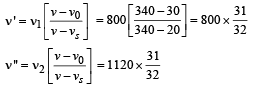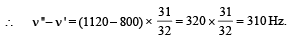Test: 35 Year JEE Previous Year Questions: Waves - Question 7

Length of a string tied to two rigid supports is 40 cm. Maximum length (wavelength in cm) of a stationary wave  produced on it is

Detailed Solution for Test: 35 Year JEE Previous Year Questions: Waves - Question 7

This will happen for fundamental mode of vibration as shown in the figure. S1 and S2 are rigid support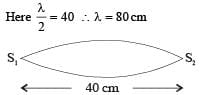Test: 35 Year JEE Previous Year Questions: Waves - Question 8

Tube A has both ends open while tube B has one end closed, otherwise they are identical. The ratio of fundamental frequency of tube A and B is

Detailed Solution for Test: 35 Year JEE Previous Year Questions: Waves - Question 8

KEY CONCEPT : The fundamental frequency for closed organ pipe is given by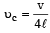and

For open organ pipe is given by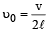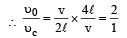Test: 35 Year JEE Previous Year Questions: Waves - Question 9

A tuning fork arrangement (pair) produces 4 beats/sec with one fork of frequency 288 cps. A little wax is placed on the unknown fork and it then produces 2 beats/sec. The frequency of the unknown fork is

Detailed Solution for Test: 35 Year JEE Previous Year Questions: Waves - Question 9

A tuning fork produces 4 beats/sec with another tuning fork of frequency 288 cps. From this information we can conclude that the frequency of unknown fork is 288 + 4 cps or 288 – 4 cps i.e. 292 cps or 284 cps. When a little wax is placed on the unknown fork, it produces 2 beats/sec. When a little wax is placed on the unknown fork, its frequency decreases and simultaneously the beat frequency decreases confirming that the frequency of the unknown fork is 292 cps.

Test: 35 Year JEE Previous Year Questions: Waves - Question 10

A wave y = a sin(ωt–kx) on a string meets with another wave producing a node at x = 0. Then the equation of the unknown wave is

Detailed Solution for Test: 35 Year JEE Previous Year Questions: Waves - Question 10

To form a node there should be superposition of this wave with the reflected wave. The reflected wave should travel in opposite direction with a phase change of π. The equation of the reflected wave will be
y = a sin (ωt + kx  + π)
⇒ y = – a sin (ωt + kx)

Test: 35 Year JEE Previous Year Questions: Waves - Question 11

When temperature increases, the frequency of a tuning fork

Detailed Solution for Test: 35 Year JEE Previous Year Questions: Waves - Question 11

KEY CONCEPT : The frequency of a tuning fork is given by the expression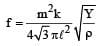As temperature increases, ℓ increases and therefore f decreases.

Test: 35 Year JEE Previous Year Questions: Waves - Question 12

The displacement y of a wave travelling in the x -direction is given by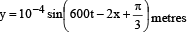where x is expressed in metres and t in seconds. The speed of the wave - motion, in ms-1 , is

Detailed Solution for Test: 35 Year JEE Previous Year Questions: Waves - Question 12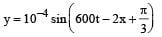But y = A sin (ωt - kx + φ)

On comparing we get ω = 600;   k = 2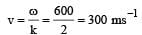Test: 35 Year JEE Previous Year Questions: Waves - Question 13

A metal wire of linear mass density of 9.8 g/m is stretched with a tension of 10 kg-wt between two rigid supports 1 metre apart. The wire passes at its middle point between the poles of a permanent magnet, and it vibrates in resonance when carrying an alternating current of frequency n. The frequency  n of the alternating source is

Detailed Solution for Test: 35 Year JEE Previous Year Questions: Waves - Question 13

KEY CONCEPT : For a string vibrating between two rigid support, the fundamental  frequency is given by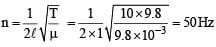As the string is vibrating in resonance to a.c of frequency n, therefore both the frequencies are same.

Test: 35 Year JEE Previous Year Questions: Waves - Question 14

A tuning fork of  known frequency 256 Hz makes 5 beats per second with the vibrating  string of a piano. The beat frequency decreases to 2 beats per second when the tension in the piano string is slightly increased. The frequency of the piano string before increasing the tension was

Detailed Solution for Test: 35 Year JEE Previous Year Questions: Waves - Question 14

A tuning fork of frequency 256 Hz  makes 5  beats/ second with the vibrating string of a piano. Therefore the frequency of the vibrating string of piano is (256 ± 5) Hz ie  either 261Hz or 251 Hz. When the tension in the piano string increases, its frequency will increases. Now since the beat frequency decreases, we can conclude that the frequency of piano string is 251Hz

Test: 35 Year JEE Previous Year Questions: Waves - Question 15

The displacement y of a particle in a medium can be expressed as,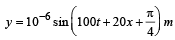where t is in second and x in meter. The speed of the wave is

Detailed Solution for Test: 35 Year JEE Previous Year Questions: Waves - Question 15

From equation given,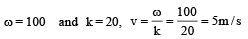Test: 35 Year JEE Previous Year Questions: Waves - Question 16

When two tuning forks (fork 1 and fork 2) are sounded simultaneously, 4 beats per second are heard. Now, some tape is attached on the prong of the fork 2. When the tuning forks are sounded again, 6 beats per second are heard. If the frequency of fork 1 is 200 Hz, then what was the original frequency of fork 2?

Detailed Solution for Test: 35 Year JEE Previous Year Questions: Waves - Question 16

No. of beats heard when fork 2 is sounded with fork 1 = Δn = 4
Now we know that if on loading (attaching tape) an unknown fork, the beat frequency increases (from 4 to 6 in this case) then the frequency of the unknown fork 2 is given by,
n = n0 - Δn = 200 – 4 = 196 Hz

Test: 35 Year JEE Previous Year Questions: Waves - Question 17

An observer moves towards a stationary source of sound, with a velocity one-fifth of the velocity of sound.What is the percentage increase in the apparent frequency ?

Detailed Solution for Test: 35 Year JEE Previous Year Questions: Waves - Question 17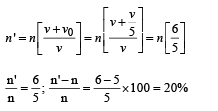Test: 35 Year JEE Previous Year Questions: Waves - Question 18

A whistle producing sound waves of frequencies 9500 HZ and above is approaching a stationary person with speed v ms–1. The velocity of  sound in air is 300 ms–1. If the person can hear frequencies upto a maximum of 10,000 HZ, the maximum value of v upto which he can hear whistle is

Detailed Solution for Test: 35 Year JEE Previous Year Questions: Waves - Question 18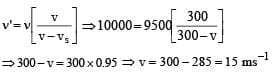Test: 35 Year JEE Previous Year Questions: Waves - Question 19

cm. It is observed to have resonant frequencies of 420 Hz and 315 Hz. There are no other resonant frequencies between these two. Then, the lowest resonant frequency for this string is

Detailed Solution for Test: 35 Year JEE Previous Year Questions: Waves - Question 19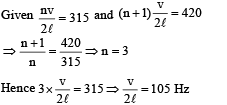Lowest resonant frequency is when  n = 1
Therefore lowest resonant frequency = 105 Hz.

Test: 35 Year JEE Previous Year Questions: Waves - Question 20

A sound absorber attenuates the sound level by 20 dB. The intensity decreases by a factor of

Detailed Solution for Test: 35 Year JEE Previous Year Questions: Waves - Question 20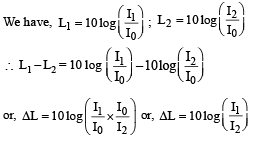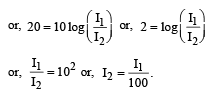⇒ Intensity decreases by a factor 100.

Test: 35 Year JEE Previous Year Questions: Waves - Question 21

While measuring the speed of sound by perfor ming a resonance column experiment, a student gets the first resonance condition at a column length of 18 cm during winter. Repeating the same experiment during summer, she measures the column length to be x cm for the second resonance. Then

Detailed Solution for Test: 35 Year JEE Previous Year Questions: Waves - Question 21

For first resonant length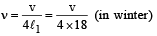For second resonant length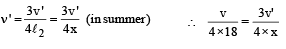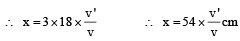v' > v  because velocity of light is greater in summer as compared to winter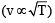∴ x > 54 cm

Test: 35 Year JEE Previous Year Questions: Waves - Question 22

A wave travelling along the x-axis is described by the equation y(x, t) = 0.005 cos (αx –βt). If the wavelength and the time period of the wave are 0.08 m and 2.0s, respectively, then α and β in appropriate units are

Detailed Solution for Test: 35 Year JEE Previous Year Questions: Waves - Question 22

y(x, t) = 0.005 cos (αx -βt) (Given)
Comparing it with the standard equation of wave y(x, t) = a cos (kx -ωt) we get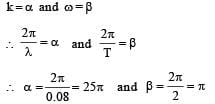Test: 35 Year JEE Previous Year Questions: Waves - Question 23

Three sound waves of equal amplitudes have frequencies (v –1), v, (v + 1). They superpose to give beats. The number of beats produced per second will be :

Detailed Solution for Test: 35 Year JEE Previous Year Questions: Waves - Question 23

Maximum number of beats = (v + 1) – (v – 1) = 2

Test: 35 Year JEE Previous Year Questions: Waves - Question 24

A motor cycle starts from rest and accelerates along a straight path at 2m/s2. At the starting point of the motor cycle there is a stationary electric siren. How far has the motor cycle gone when the driver hears the frequency of the siren at 94% of its value when the motor cycle was at rest? (Speed of sound = 330 ms–1)

Detailed Solution for Test: 35 Year JEE Previous Year Questions: Waves - Question 24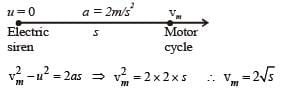According to Doppler’s effect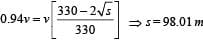Test: 35 Year JEE Previous Year Questions: Waves - Question 25

The equation of a wave on a string of linear mass density 0.04 kg m–1 is given by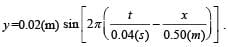The tension in the string is

Detailed Solution for Test: 35 Year JEE Previous Year Questions: Waves - Question 25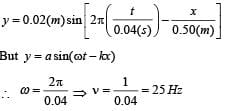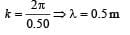∴ velocity, v = nλ = 25 × 0.5 m/s = 12.5 m/s
Velocity on a string is given by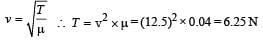Test: 35 Year JEE Previous Year Questions: Waves - Question 26

The transverse displacement y (x, t) of a wave on a string is given by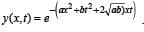. This represents a:

Detailed Solution for Test: 35 Year JEE Previous Year Questions: Waves - Question 26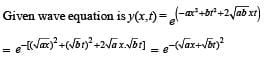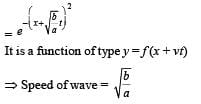Test: 35 Year JEE Previous Year Questions: Waves - Question 27

A cylindrical tube, open at both ends, has a fundamental frequency, f, in air. The tube is dipped vertically in water so that half of it is in water. The fundamental frequency of the air-column is now :

Detailed Solution for Test: 35 Year JEE Previous Year Questions: Waves - Question 27

The fundamental frequency of open tube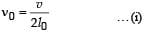That of closed pipe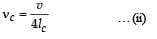According to the problem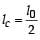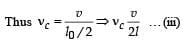From equations (i) and (iii)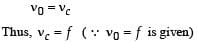Test: 35 Year JEE Previous Year Questions: Waves - Question 28

A sonometer wire of length 1.5 m is made of steel. The tension in it produces an elastic strain of 1%. What is the fundamental frequency of steel if density and elasticity of steel are 7.7 × 103 kg/m3 and 2.2 × 1011 N/m2 respectively ?

Detailed Solution for Test: 35 Year JEE Previous Year Questions: Waves - Question 28

Fundamental frequency,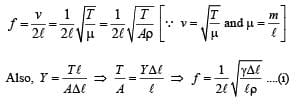Putting the value of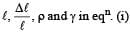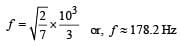Test: 35 Year JEE Previous Year Questions: Waves - Question 29

A pipe of length 85 cm is closed from one end. Find the number of possible natural oscillations of air column in the pipe whose frequencies lie below 1250 Hz. The velocity of sound in air is 340 m/s.

Detailed Solution for Test: 35 Year JEE Previous Year Questions: Waves - Question 29

Length of pipe = 85  cm = 0.85m Frequency of oscillations of air column in closed organ pipe is given by,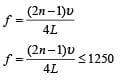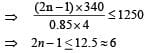Test: 35 Year JEE Previous Year Questions: Waves - Question 30

A train is moving on a straight track with speed 20 ms–1. It is blowing its whistle at the frequency of 1000 Hz. The percentage change in the frequency heard by a person standing near the track as the train passes him is (speed of sound = 320 ms–1) close to :

Detailed Solution for Test: 35 Year JEE Previous Year Questions: Waves - Question 30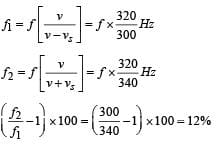Test: 35 Year JEE Previous Year Questions: Waves - Question 31

A uniform string of length 20 m is suspended from a rigid support. A short wave pulse is introduced at its lowest end. It starts moving up the string. The time taken to reach the supports is :

(take g = 10 ms–2)

Detailed Solution for Test: 35 Year JEE Previous Year Questions: Waves - Question 31

We know that velocity in string is given by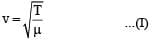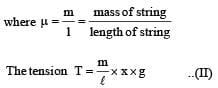From (a) and (b)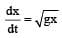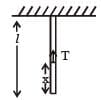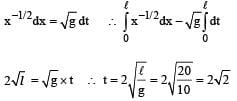Test: 35 Year JEE Previous Year Questions: Waves - Question 32

A pipe open at both ends has a fundamental frequency f in air. The pipe is dipped vertically in water so that half of it is in water. The fundamental frequency of the air column is now :

Detailed Solution for Test: 35 Year JEE Previous Year Questions: Waves - Question 32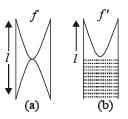The fundamental  frequency in case (a) is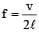The fundamental frequency in case (b) is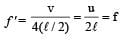## Physics For JEE

258 videos|633 docs|255 tests
Information about Test: 35 Year JEE Previous Year Questions: Waves Page
In this test you can find the Exam questions for Test: 35 Year JEE Previous Year Questions: Waves solved & explained in the simplest way possible. Besides giving Questions and answers for Test: 35 Year JEE Previous Year Questions: Waves, EduRev gives you an ample number of Online tests for practice

## Physics For JEE

258 videos|633 docs|255 tests(Scan QR code)